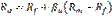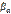# Capital Asset Pricing Model (CAPM)

The capital asset pricing model (CAPM) Building on the earlier work of Harry Markowitz on diversification and modern portfolio theory, the capital asset pricing model (CAPM) was introduced by Jack Treynor (1961, 1962), William Sharpe (1964), John Lintner (1965) and Jan Mossin (1966) independently. Sharpe, Markowitz and Merton Miller jointly received the Nobel Memorial Prize in Economics for this contribution to the field of financial economics.

The general idea behind CAPM is that investors need to be compensated in two ways: time value of money and risk. The time value of money is represented by the risk-free (rf) rate. The market risk premium compensates the investor needs for taking on additional risk is calculated by taking a risk measure (beta) that compares the returns of the asset to the market over a period of time and to the market premium.Whereis risk-free rate,Rm – expected market return,- the beta of asset.

Beta is the sensitivity of the expected excess asset returns to the expected excess market returns.The Security Market Line graphs the results from the capital asset pricing model (CAPM) formula. The x-axis represents the risk (beta), and the y-axis represents the expected return. The market risk premium is determined from the slope of the SML.

The relationship between β and required return is plotted on the securities market line (SML), which shows expected return as a function of β. The intercept is the nominal risk-free rate available for the market, while the slope is the market premium, E(Rm)− Rf. The securities market line can be regarded as representing a single-factor model of the asset price, where Beta is exposure to changes in value of the Market. The equation of the SML is thus:

It is a useful tool in determining if an asset being considered for a portfolio offers a reasonable expected return for risk. Individual securities are plotted on the SML graph. If the security's expected return versus risk is plotted above the SML, it is undervalued since the investor can expect a greater return for the inherent risk. And a security plotted below the SML is overvalued since the investor would be accepting less return for the amount of risk assumed.

 Дата добавления: 2015-09-04; просмотров: 485. Нарушение авторских прав; Мы поможем в написании вашей работы!
Studopedia.info - Студопедия - 2014-2022 год . (0.017 сек.) русская версия | украинская версия